### 三、    曲面积分

[对曲面面积的曲面积分]

1° S为逐片光滑的双侧曲面*

z=z(x,y)   ((x,y))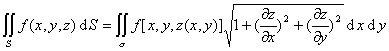2° 若曲面S由连续可微函数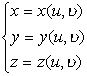((u,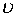)Ω)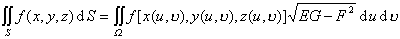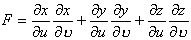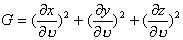* 曲面上某一点的法线方向的选定，唯一确定了曲面上所有其他点的法线方向，它们就是选定方向的

[对坐标的曲面积分]  S为光滑的双侧曲面，为它的正面，即由法线方向n(cosα, cosβ,cosγ)所确定的一侧，P=P(x,y,z),Q=Q(x,y,z),R=R(x,y,z)为在曲面S上有定义并且连续的函数，则若曲面S由连续可微函数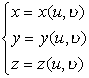((u,)Ω)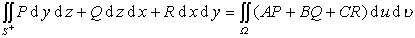[斯托克斯公式]  C是包围逐片光滑有界双侧曲面S的逐段光滑简单闭曲线，P=P(x,y,z),Q=Q(x,y,z),R=R(x,y,z)是在S+C上连续可微函数，则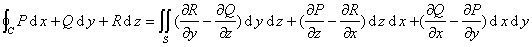[高斯公式]  S为包含体积V的逐片光滑曲面P=P(x,y,z),Q=Q(x,y,z),R=R(x,y,z)及其一阶偏导数在V+S上连续，则有高斯公式：